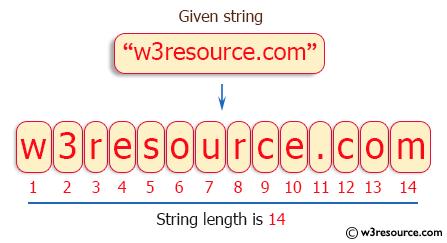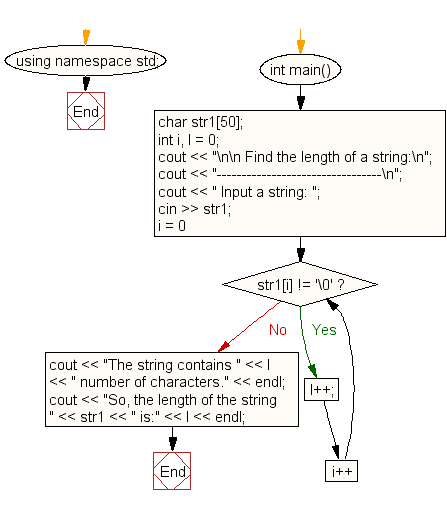﻿ C++ : Find the length of a string without library function# C++ Exercises: Find the length of a string without using the library function

## C++ For Loop: Exercise-34 with Solution

Write a program in C++ to find the length of a string without using the library function.

Pictorial Presentation:Sample Solution:-

C++ Code :

``````#include <iostream>
#include <string>
using namespace std;

int main()
{
char str1;
int i, l = 0;
cout << "\n\n Find the length of a string:\n";
cout << "---------------------------------\n";
cout << " Input a string: ";
cin >> str1;
for (i = 0; str1[i] != '\0'; i++) {
l++;
}
cout << "The string contains " << l << " number of characters." << endl;
cout << "So, the length of the string " << str1 << " is:" << l << endl;
}
``````

Sample Output:

``` Find the length of a string:
---------------------------------
Input a string: w3resource.com
The string contains 14 number of characters.
So, the length of the string w3resource.com is:14
```

Flowchart:C++ Code Editor:

Contribute your code and comments through Disqus.

What is the difficulty level of this exercise?

﻿